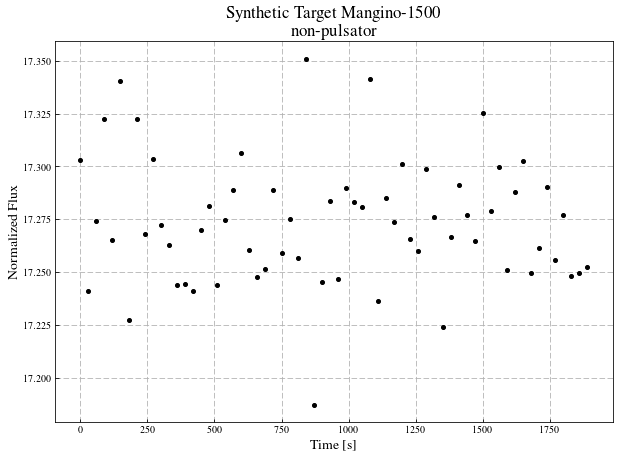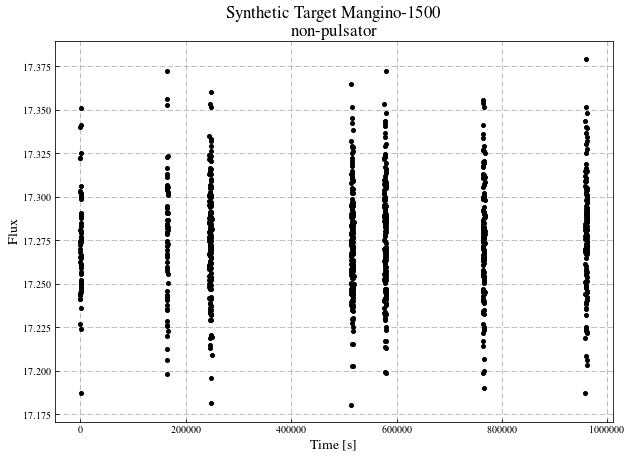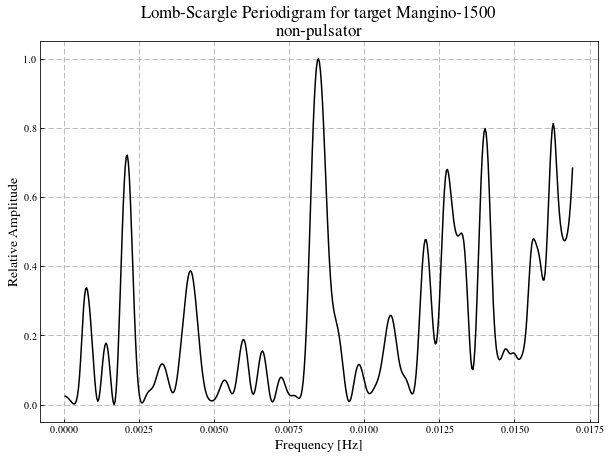Synthetic Data Generation To Analysis

# Aim¶

This notebook will provide a complete record of the steps used to generate sythetic data, construct a network, train the network, and validate the effectivness of that network. Hopefully by following cell by cell the procedure will be reproducable by any who are interested.

The Structure of this network will be as follows: Code will be presented, the following cell will provide an explanation of the code if that is nessesairy.

## Creating Synthetic Data¶

In :
from astroSynth import POS, PVS


POS provide the basic object of astroSynth, POS can create many targets with one $\rightarrow$ many light curves each. POS also provides the foundation by which to quickly query these light curves and their assosicated Lomb-Scargle periodigrams

In :
dI = PVS()
dII = POS()
dI.load(directory='d-I')
dII.load(directory='d-II')

Item Loc:   0%|          | 0/1000 [00:00<?, ?it/s]
Item Loc: 100%|██████████| 1000/1000 [00:00<00:00, 41575.92it/s]
Item Ref:   0%|          | 0/100000 [00:00<?, ?it/s]
Item Ref:  16%|█▌        | 15782/100000 [00:00<00:00, 156660.41it/s]
Item Ref:  44%|████▍     | 43881/100000 [00:00<00:00, 180102.93it/s]
Item Ref:  72%|███████▏  | 71639/100000 [00:00<00:00, 201310.73it/s]
Item Ref: 100%|██████████| 100000/100000 [00:00<00:00, 248599.08it/s]
Object Class:   0%|          | 0/100000 [00:00<?, ?it/s]
Object Class:  60%|█████▉    | 59812/100000 [00:00<00:00, 598114.87it/s]
Object Class: 100%|██████████| 100000/100000 [00:00<00:00, 589953.51it/s]
Object Meta:   0%|          | 0/7 [00:00<?, ?it/s]
Object Meta: 100%|██████████| 7/7 [00:00<00:00, 114688.00it/s]

# IF YOU HAVE ALREADY RUN THE INSTANTIATE, BUILD, GENERATE, AND SAVE CODE RUN THESE COMMANDS TO LOAD THE PREVIOUSLY BUILT DATA AND SAVE YOURSELF SOME TIME¶

In :
dI = PVS(Number=100000, name='d-I', noise_range=[0.001, 0.045])
dII = POS(number=100000, noise_range=[0.001, 0.045], numpoints=100000, name='d-II')


Here we generate the POS objects, parameters are as follows:

number: the number of objects to generate

noise_range: the range of noise values to pick from when inserting noise into the light curve

numpoints: the number of total exposure times from t=t0 to t=tf (there will be far fewer points in the final light curves if visit number > 1 as points in the time between visits will no longer be present)

name: name the survey will take when saved to disk

We create 2 surveys, _S and _V, in order that we might analyze data with single light curves per target and data with multiple light curves per target. _S will have single light curves per target and _V will have multiple light curves per target.

In [ ]:
dI.build(amp_range=[0, 0.02], freq_range=[0.0008333, 0.01667], L_range=[1, 3])
dII.build(amp_range=[0, 0.02], freq_range=[0.0008333, 0.01667], visits=[1, 50], L_range=[1, 3])

dI.generate(pfrac=0.5)
dII.generate(pfrac=0.5)

dI.save()
dII.save()


Next we generate the light curves. This is the most computationally expensive part of this section ($\sim 4.5$ hours on a 2016 mobile class intel i7 running at $\sim2.5 GHz$). We also spesify the fraction of targets that will be pulsators here in decimal. 0.5 is choosen so as to limit any sample biases that the network might pick up latter on.

Finally we save the Light Curve data to directories named the same name as the survey. Once you run this command you can load the data into a POS object at any time in the future as follows:

from astroSynth import POS
survey = POS()
survey.load(directory='saved_survey')


where "saved_survey" is the name which was assigned to the POS object before it was saved. In this case that would be "dI" and "dII".

Again Note that this command can take a good amount of time ($\sim 15$ minutes) as it needs to write a lot of data to disk.

In :
from matplotlib.ticker import MultipleLocator, FormatStrFormatter
import matplotlib.pyplot as plt
from matplotlib import rcParams
import matplotlib as mpl
mpl.rc("savefig", dpi=150)
mpl.rc('font',**{'family':'serif','serif':['Times']})
mpl.rc('text', usetex=True)
rcParams['xtick.direction'] = 'in'
rcParams['ytick.direction'] = 'in'
%matplotlib inline

In :
target = 2
visit = 0

In :
# Plot one visit from a target

plt.figure(figsize=(10, 7))
plt.plot(dII[target][visit], dII[target][visit], 'ko', markersize=4)
plt.ylabel('Normalized Flux', fontsize=14)
plt.xlabel('Time [s]', fontsize=14)
if dII[target][visit] == 1:
pul = 'pulsator'
elif dII[target][visit] == 0.0:
pul = 'non-pulsator'
plt.title('Synthetic Target {}\n{}'.format(dII[target].name, pul), fontsize=17)
plt.grid(linestyle='--')
plt.show()
plt.close()

# Plot the entirety of a targets light curve
plt.figure(figsize=(10, 7))
light_curve = dII.get_full_lc(n=target)
plt.plot(light_curve, light_curve, 'ko', markersize=4)
plt.ylabel('Flux', fontsize=14)
plt.xlabel('Time [s]', fontsize=14)
if dII[target][visit] == 1:
pul = 'pulsator'
elif dII[target][visit] == 0.0:
pul = 'non-pulsator'
plt.title('Synthetic Target {}\n{}'.format(dII[target].name, pul), fontsize=17)
plt.grid(linestyle='--')
plt.show()
plt.close()Above we see two figures: Top -- Light Curve for the first visit of a target, Bottom -- the full light curve for a target. The visit structure is clearly visible in the full light curve.

In :
plt.figure(figsize=(10, 7))
lsp = dII.get_ft_sub(n=target, sub_element=visit, s=500)
if dII[target][visit] == 1:
pul = 'pulsator'
elif dII[target][visit] == 0.0:
pul = 'non-pulsator'
plt.plot(lsp, lsp, 'k')
plt.xlabel('Frequency [Hz]', fontsize=14)
plt.ylabel('Relative Amplitude', fontsize=14)
plt.title('Lomb-Scargle Periodigram for target {}\n{}'.format(dII[target].name, pul), fontsize=17)
plt.grid(linestyle='--')## Setting Up An Artificial Neural Network (ANN)¶

We will use the python module Keras to construct and interface with our neural networks. We use an ANN to analyze the data of independant light curves as this is can be thought of as fundamentally a 1D problem.

In :
import numpy as np
from keras.utils import np_utils
from keras.models import Sequential
from keras.layers import Dense, Activation, Dropout
from sklearn.cross_validation import train_test_split

Using TensorFlow backend.
//anaconda/envs/ML3/lib/python3.6/site-packages/sklearn/cross_validation.py:44: DeprecationWarning: This module was deprecated in version 0.18 in favor of the model_selection module into which all the refactored classes and functions are moved. Also note that the interface of the new CV iterators are different from that of this module. This module will be removed in 0.20.
"This module will be removed in 0.20.", DeprecationWarning)


For this work we made use of Google's TensorFlow modlue to form the backend of Keras, no testing was done with the other avalible backend for Keras Theano.

In :
def one_hot_encode_object_array(arr):
uniques, ids = np.unique(arr, return_inverse=True)
return np_utils.to_categorical(ids, len(uniques))

def save_model(model, name='Model'):
model_json = model.to_json()
with open(f'.json', 'w') as f:
f.write(model_json)
model.save_weights(f'.h5')
print('Saved Model to Disk')


Above are two "helper functions".

one_hot_encode_object_array preforms a one hot encoding on based on possible classification. That is to say that if there are two possible states that each data entry could fall into, A and B, and we had a vector of the form [A, B, A, A, A, B, A,...,B, B, A, B, B]$^$. Then preforming a one hot encode would return a vector of the form [[1, 0], [0, 1], [1, 0], [1, 0], [1, 0], [0, 1], [1, 0],...,[0, 1], [0, 1], [1, 0], [0, 1], [0, 1]]$^$. This one hot encoding is essetial for a classification network to operate.

The Second Function simply serialized and saves the model to a JSON file so that it may be accesed in future. If you do not have python 3.6 or greater installed then this function will not run properly due to its use of f-strings. To fix this change any strings prefeaced with f to one with format, so for example

with open(f'.json', 'w') as f:


would become

with open('.json'.format(name=name), 'w') as f:

In :
def build_model(af='relu'):
model = Sequential()
model.add(Dense(500, input_dim=503))
model.add(Activation(af))
model.add(Dropout(0.2))
model.add(Dense(500))
model.add(Activation(af))
model.add(Dropout(0.2))
model.add(Dense(2))
model.add(Activation('softmax'))

model.compile(loss='categorical_crossentropy', optimizer='adam', metrics=['accuracy'])

return model

model = build_model()


Here we build the structure of the ANN. I will walk threw each line

1. Defining the network as a linear set of layers
2. Add a fully connected layer with 503 inputs and 1000 outputs [INPUT]
• We will pass into the network the full Lomb-Scarge Periodigram (LSP) which will be forced to have 500 frequency bins, as well as 3 parameters spesifically extracted from the LSP
1. The maximum amplitude in the LSP
2. The median value of the LSP
3. The freququency of the maximum amplitude in the LSP
3. Add the layer to apply the activation function to the outputs of the previous layer
• We use the rectified linear unit as an activation function.
4. Apply a 20 percent dropout to the network here
5. Same as layer 2 [HIDDEN]
6. Same as layer 3
7. Same as layer 5
8. Add an two value output layer [OUTPUT]
9. Apply the softmax activation function to the outputs to scale them between 0-1 in probability space.
10. We now compile the model together
• loss defines how the network should measure its sucsess, as we aim to classify data entries into catagories we use catagorical_crossentropy
• the optimizer defines how the model should modify itself, adam is a common omptimizer that provides adaquet results
• metrics tells the network what we would like it to report, here we want accuracy which will be returened as a decimal percentage.

Once the function is defined to build the network the network is instantiated

## Using an ANN¶

In :
from tqdm import tqdm
from hyperas.distributions import uniform


Progress bar module, if you installed astroSynth you have tqdm installed, we also import a module to tune hyperparameters, While this is not used here as they have been tuned prior to the rease of this notebook

In :
#DELETE THIS FUNCTION BEFORE SUBMISSION
def deal_with_detrad(freq, amp, Class):
for i, (f, a, c) in enumerate(zip(freq, amp, Class)):
fmp = f[a.index(max(a))]
if 2e-5-fmp <= 0.008386 <= 2e-5+fmp and c == 1:
Class[i] = 0
return Class

run_length = int(dI.size/(dI.size/10))-2
for i, (Freq, Amp, Class, N) in tqdm(enumerate(dI.batch_get(batch_size=int(dI.size/10),
ft=True, s=500)), total=run_length):
if i >= run_length:
break

Xt = list()
for x in Amp:
Xtt = [j/max(x) for j in x]
Xt.append(Xtt)

Freq
X = np.array(Xt)
Y = deal_with_detrad(Freq, Amp, Class)
X = np.insert(X, 0, [Freq[k][x.argmax()] for k, x in enumerate(X)], axis=1)   # Frequency of Max Amplitude
X = np.insert(X, 0, [max(x) for x in Amp], axis=1)                            # Max Amplitude
X = np.insert(X, 0, [np.median(x) for x in Amp], axis=1)                      # Median Value of LSP

Y = one_hot_encode_object_array(Y)

model.fit(X, Y, verbose=1, nb_epoch=5, batch_size=100)

  0%|          | 0/8 [00:00<?, ?it/s]
//anaconda/envs/ML3/lib/python3.6/site-packages/keras/models.py:844: UserWarning: The nb_epoch argument in fit has been renamed epochs.
warnings.warn('The nb_epoch argument in fit '

Epoch 1/5
10000/10000 [==============================] - 1s - loss: 0.4925 - acc: 0.7858
Epoch 2/5
10000/10000 [==============================] - 0s - loss: 0.4353 - acc: 0.8162
Epoch 3/5
10000/10000 [==============================] - 0s - loss: 0.4046 - acc: 0.8320
Epoch 4/5
10000/10000 [==============================] - 0s - loss: 0.3925 - acc: 0.8360
Epoch 5/5
10000/10000 [==============================] - 0s - loss: 0.3805 - acc: 0.8452     
 12%|█▎        | 1/8 [01:17<09:03, 77.66s/it]
Epoch 1/5
10000/10000 [==============================] - 0s - loss: 0.3960 - acc: 0.8378
Epoch 2/5
10000/10000 [==============================] - 0s - loss: 0.3807 - acc: 0.8435
Epoch 3/5
10000/10000 [==============================] - 0s - loss: 0.3721 - acc: 0.8459
Epoch 4/5
10000/10000 [==============================] - 0s - loss: 0.3667 - acc: 0.8518
Epoch 5/5
10000/10000 [==============================] - 0s - loss: 0.3584 - acc: 0.8538     
 25%|██▌       | 2/8 [02:23<07:24, 74.08s/it]
Epoch 1/5
10000/10000 [==============================] - 0s - loss: 0.3770 - acc: 0.8479
Epoch 2/5
10000/10000 [==============================] - 0s - loss: 0.3700 - acc: 0.8480
Epoch 3/5
10000/10000 [==============================] - 0s - loss: 0.3555 - acc: 0.8554
Epoch 4/5
10000/10000 [==============================] - 0s - loss: 0.3534 - acc: 0.8566
Epoch 5/5
10000/10000 [==============================] - 0s - loss: 0.3438 - acc: 0.8586     
 38%|███▊      | 3/8 [03:29<05:58, 71.70s/it]
Epoch 1/5
10000/10000 [==============================] - 0s - loss: 0.3751 - acc: 0.8487
Epoch 2/5
10000/10000 [==============================] - 0s - loss: 0.3702 - acc: 0.8491
Epoch 3/5
10000/10000 [==============================] - 0s - loss: 0.3624 - acc: 0.8538
Epoch 4/5
10000/10000 [==============================] - 0s - loss: 0.3583 - acc: 0.8528
Epoch 5/5
10000/10000 [==============================] - 0s - loss: 0.3486 - acc: 0.8576     
 50%|█████     | 4/8 [04:34<04:39, 69.81s/it]
Epoch 1/5
10000/10000 [==============================] - 0s - loss: 0.3571 - acc: 0.8536
Epoch 2/5
10000/10000 [==============================] - 0s - loss: 0.3424 - acc: 0.8620
Epoch 3/5
10000/10000 [==============================] - 0s - loss: 0.3391 - acc: 0.8635
Epoch 4/5
10000/10000 [==============================] - 0s - loss: 0.3322 - acc: 0.8658
Epoch 5/5
10000/10000 [==============================] - 0s - loss: 0.3248 - acc: 0.8671     
 62%|██████▎   | 5/8 [05:41<03:26, 68.70s/it]
Epoch 1/5
10000/10000 [==============================] - 0s - loss: 0.3660 - acc: 0.8535
Epoch 2/5
10000/10000 [==============================] - 0s - loss: 0.3544 - acc: 0.8539
Epoch 3/5
10000/10000 [==============================] - 0s - loss: 0.3472 - acc: 0.8596
Epoch 4/5
10000/10000 [==============================] - 0s - loss: 0.3435 - acc: 0.8612
Epoch 5/5
10000/10000 [==============================] - 0s - loss: 0.3427 - acc: 0.8620     
 75%|███████▌  | 6/8 [06:48<02:16, 68.46s/it]
Epoch 1/5
10000/10000 [==============================] - 0s - loss: 0.3566 - acc: 0.8562
Epoch 2/5
10000/10000 [==============================] - 0s - loss: 0.3442 - acc: 0.8645
Epoch 3/5
10000/10000 [==============================] - 0s - loss: 0.3363 - acc: 0.8654
Epoch 4/5
10000/10000 [==============================] - 0s - loss: 0.3307 - acc: 0.8703
Epoch 5/5
10000/10000 [==============================] - 0s - loss: 0.3237 - acc: 0.8687     
 88%|████████▊ | 7/8 [07:53<01:07, 67.28s/it]
Epoch 1/5
10000/10000 [==============================] - 0s - loss: 0.3632 - acc: 0.8544
Epoch 2/5
10000/10000 [==============================] - 0s - loss: 0.3427 - acc: 0.8615
Epoch 3/5
10000/10000 [==============================] - 0s - loss: 0.3465 - acc: 0.8603
Epoch 4/5
10000/10000 [==============================] - 0s - loss: 0.3326 - acc: 0.8650
Epoch 5/5
10000/10000 [==============================] - 0s - loss: 0.3239 - acc: 0.8705     
100%|██████████| 8/8 [08:56<00:00, 66.02s/it]

Here we see the training of the network, I will go line by line:

1. Defining the run length both so tqdm knows how large to draw the progress bar but also so that if one wants to run a subset of training they can, simply by changing that number.
• I subtract one so that there will be some data the network has never seen, which we will latter use to validate the preformance of the network.
2. Open the for loop, an index value is gained through the use of an enumerate enviroment, and the returned tuple from PVS().batch_get is expanded. Here we define the size of the returned batch, tell it that we want fts (LSPs) returned (as opposed to light curves). And finally provided the number of frequency bins per ft.
3. Check if the run length has been exceded.
4. if run length is exceded break the loop.
5. Take the amplitude list returned from PVS().batch_get() and cast it to a numpy array [X]
6. Insert into the 0th position of X the frequency of the maximum amplitude in the ft
7. Insert into the 0th position of X the maximum amplitude in the ft
8. Insert into the 0th position of X the medaian amplitude of the ft
9. run one hot encoding on the class list returned from PVS().batch_get() [Y]
10. Train the model on the input data, X, against the classification data, Y.
• verbose = 1 provids the user with a progress bar at each trainish epoch
• nb_epochs = n will train the network on the same data set n times, generally a balance must be found so that n if large enough to let the netowrk learn the datas features effectivly, but not so high that the network overfits the data
In :
start = 80000
stop = 98000

Val_Freq, Val_Amp, Val_Class, Val_N = dI.__batch_get_ft__(start=start, stop=stop, s=500)

Val_Y = one_hot_encode_object_array(Val_Class)

Val_Xt = list()
for x in Val_Amp:
Val_Xtt = [j/max(x) for j in x]
Val_Xt.append(Val_Xtt)
Val_X = np.array(Val_Xt)
Val_X = np.insert(Val_X, 0, [Val_Freq[k][x.argmax()] for k, x in enumerate(Val_X)], axis=1)
Val_X = np.insert(Val_X, 0, [max(x) for x in Val_Amp], axis=1)
Val_X = np.insert(Val_X, 0, [np.median(x) for x in Val_Amp], axis=1)


Here we build the validation data arrays. Using PVS().__batch_get_ft__() we can get a batch of fts covering a user defined range. Which we do using the start and stop parmenters. These are calculated to start where the training data left off and end at the end at the end of the data set. The rest of this cell is functionally identical to building the training data arrays as we did in the above cells.

In :
score = model.evaluate(Val_X, Val_Y, verbose=0)
print('Model Loss: {:2f}, Model Accuracy: {:2f}%'.format(score, score*100))

Model Loss: 0.483217, Model Accuracy: 75.238889%


evaluating the model on the validation data provides the loss, and whatever metrics we compiled the model with (in thsi case accuracy) to see structure of the return from model.evaluate one can run model.metrics_names.

While we now have a percentagae accuracy it would be nice to have a way of visualizing how this network classifies, as such we will plot all data points in a 2D parameter space and color them according to how the network classifies. We will also generate a second plot in the same parameter space, however this time coloring points based on what they actually are.

In :
predicted = model.predict_classes(Val_X)

17376/18000 [===========================>..] - ETA: 0s

Here we use the Sequential().predict_classes() method to generate a list of classes for the validation data

In :
save_model(model, name='ANNModel_GALEX_DatRad_Accounted_For')

Saved Model to Disk

In :
mp = [max(x) for x in Val_Amp]
mv = [np.median(x) for x in Val_Amp]

ct = Val_Class
cp = predicted

fig = plt.figure(figsize=(10, 14))
axpred = fig.add_subplot(211)
axtrue = fig.add_subplot(212)

axtrue.scatter(mv, mp, c=ct)
axpred.scatter(mv, mp, c=cp)

axtrue.set_xlim(0, 0.0010)
axtrue.set_ylim(0, 0.0035)
axpred.set_xlim(0, 0.0010)
axpred.set_ylim(0, 0.0035)

axtrue.set_title('True Classse for {} targets'.format(len(ct)), fontsize=17)
axpred.set_title('Predicted Classse for {} targets'.format(len(cp)), fontsize=17)

axtrue.set_xlabel('Median Value in LSP', fontsize=15)
axpred.set_xlabel('Median Value in LSP', fontsize=15)

axtrue.set_ylabel('Maximum Amplitude in LSP', fontsize=15)
axpred.set_ylabel('Maximum Amplitude in LSP', fontsize=15)

axtrue.grid(linestyle='--')
axpred.grid(linestyle='--')